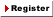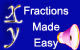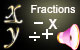New User RegisterLogin Member: Email: Password:
Math >> Arithmatic >> Mathematics >> Fraction (Arithmatic)
 Overview: Prime numbers, Greatest common factor, Least common multiple, What is a fraction?, Equivalent fractions, Comparing fractions, Converting and reducing fractions, Lowest , terms in fractions, Improper fractions, Mixed numbers, Converting mixed numbers to , improper fractions , Converting improper fractions to mixed numbers, Writing a fraction as a decimal, Rounding a fraction to the nearest hundredth, Adding and subtracting fractions, Adding and subtracting mixed numbers, Multiplying fractions and whole , numbers, Multiplying fractions and fractions, Multiplying mixed numbers, Reciprocal of a fraction, Dividing fractions, Dividing mixed numbers, Simplifying complex fractions, Repeating decimals1